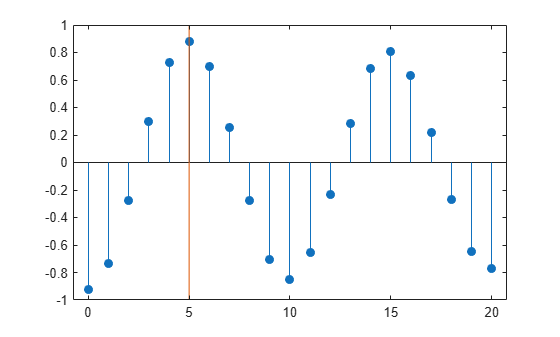## Cross-Correlation of Phase-Lagged Sine Wave

This example shows how to use the cross-correlation sequence to estimate the phase lag between two sine waves. The theoretical cross-correlation sequence of two sine waves at the same frequency also oscillates at that frequency. Because the sample cross-correlation sequence uses fewer and fewer samples at larger lags, the sample cross-correlation sequence also oscillates at the same frequency, but the amplitude decays as the lag increases.

Create two sine waves with frequencies of $2\pi /10$ rad/sample. The starting phase of one sine wave is 0, while the starting phase of the other sine wave is $-\pi$ radians. Add $N\left(0,0.2{5}^{2}\right)$ white noise to the sine wave with the phase lag of $\pi$ radians. Set the random number generator to the default settings for reproducible results.

```rng default t = 0:99; x = cos(2*pi*1/10*t); y = cos(2*pi*1/10*t-pi)+0.25*randn(size(t));```

Obtain the sample cross-correlation sequence for two periods of the sine wave (10 samples). Plot the cross-correlation sequence and mark the known lag between the two sine waves (5 samples).

```[xc,lags] = xcorr(y,x,20,'coeff'); stem(lags(21:end),xc(21:end),'filled') hold on plot([5 5],[-1 1]) ax = gca; ax.XTick = 0:5:20;```You see that the cross-correlation sequence peaks at lag 5 as expected and oscillates with a period of 10 samples.